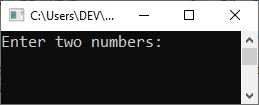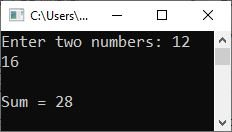# C++ typedef

C++ allows you to explicitly define new data type names by using the keyword typedef. Using typedef does not actually create a new data class; rather, it defines a new name for an existing type. This can increase the portability of a program, as only the typedef statements would have to be changed.

Using typedef can also help your code document itself by giving the standard data types names that are easy to understand.

## C++ typedef Syntax

The general form of the "typedef" keyword in C++ is as follows:

`typedef type name;`

where "type" is any C++ data type, and "name" is the new name for this type. This defines another name for the standard type of C++. For example, you would create a new name for the "float" type by using the following statement:

`typedef float realNum;`

A new name for the type float has been created through typedef. Now, when we write "realNum" in the program, it refers to "float." That is, the above statement tells the compiler to recognize "realNum" as an alternative name for "float."

You can now use realNum to create float variables. For example:

`realNum loan, saving, installment;`

loan, saving, and installment are variables of type realNum, which is another name for float. Thus, loan, saving, and installment are all float variables.

Note: Remember that typedef does not create any new data types but rather provides an alternative name for standard types. Reference provides an alias name for a variable, and typedef provides an alias name for a data type. Let's take an example, demonstrating typedef in C++.

## C++ typedef Example

Here's an example program that demonstrates typedef in C++.

```#include<iostream>
using namespace std;

int main()
{
typedef int integer;
integer a, b, x;

cout<<"Enter two numbers: ";
cin>>a>>b;
x = a+b;

cout<<"\nSum = "<<x;
cout<<endl;

return 0;
}```

The following snapshot shows the initial output produced by the above example program:Now type a number, say 12, and hit the ENTER key. Then type another number, say 16, and hit the ENTER key again to produce the following output:However, you can also use the original name of the data type even if that data type is already renamed in the program, using the typedef keyword. As an example:

```#include<iostream>
using namespace std;

int main()
{
typedef int integer;
integer a, b;
int x;

a = 10, b = 20;
x = a + b;

cout<<"Sum = "<<x;
cout<<endl;

return 0;
}```

The output should be:

`Sum = 30`

Let's take another program for a complete understanding of typedef in C++. This program uses typedef to create another name (integer) for int and uses this name to create another name (integer_type) for the same data type, which is int. Again, using this name, it creates a new name (integer_data_type) for the data type int using the typedef. The names are integer, integer_type, and then integer_data_type; all three names are used to create variables of type int, as shown in this C++ program:

```#include<iostream>
using namespace std;

int main()
{
typedef int integer;
integer a;

typedef integer integer_type;
integer_type b;

typedef integer_type integer_data_type;
integer_data_type x;

a = 10, b = 20;
x = a + b;

cout<<"Sum = "<<x;
cout<<endl;

return 0;
}```

You will get the exact same output as the previous program's output.

C++ Quiz

« Previous Tutorial Next Tutorial »